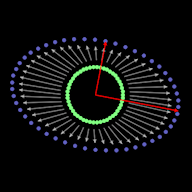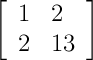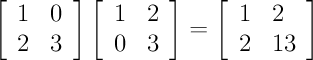metamerist

Saturday, March 29, 2008

I Love the Unknown

Last night, we watched and enjoyed Rocket Science, written and directed by Jeffrey Blitz who also directed the excellent documentary Spellbound. The film has the sort of quirky Andersonian dryness I enjoy. Anna Kendrick brings the best overachieving high school student since Reece Witherspoon's Election character, Tracy Flick.

Artist: Clem Snide
Song: I Love the Unknown (mp3)

Tuesday, March 25, 2008

Miss Peggy Guy

The incomparable talents of Miss Peggy Guy...

Matrices & GeometryIf you're searching for an applet that shows properties and effects of a 2D matrix, look no further...
My latest creation: matrix action applet

Saturday, March 22, 2008

Googlaziness & Cholesky 2x2

This morning I spent more time than I should have searching the Internet for a means of calculating the Cholesky decomposition of a 2x2 matrix. Frustrated with a lack of sufficiently instant satisfaction, I got off my mental posterior and decided to figure it out for myself--a point after which I quickly realized it was easy. Maybe this post will make it easy for you to find the answer in Google, so you can be Googlazy, but I encourage you to first take a stab at figuring it out on your own.

"Cholesky decomposition" sounds so ominous. A sad fact with linear algebra (and it is not alone in mathematics in this respect) is things often sound much more complicated and mind-numbing than they really are. All we're talking about with Cholesky is a factorization that's very similar to the square root of a square matrix.

Cholesky is typically shown as:

L * LT = A

The L matrix is lower triangular, which means all the cells above and to the right of the diagonal are zero. The 'T' indicates the transpose of L, which will be upper triangular because all of the values are mirrored about the diagonal.

In the 2x2 case, let's plug in some variables for our unknowns, multiply it all out and see what we get:It's clear from that not every 2x2 matrix is going to have a Cholesky decomposition consisting of real numbers.

Why?

First we need a real root for the cell in the upper-left corner (a2). If the upper-left cell is non-negative, we can calculate its square root and get 'a'.

Another thing that's clear is your matrix needs to be symmetric for Cholesky. Why? Because we have 'ab' in the lower-left and the 'ab' in upper-right. If your matrix isn't symmetric, looks like it's time to give up on this. But if it is, you can easily calculate 'b' by dividing by 'a' (which we know because we figured it out in the first step).

After we've successfully calculated 'a' and 'b', all that remains is 'c'. Since the lower-right corner is equal to 'b2+c2', we can calculate the square root of the lower-right value after subtracting 'b' squared.

You may be wondering what will happen if b2 is greater than c2. The answer is 'That will be bad!' Not 'don't cross the streams' bad--just 'You won't get a real number' bad.

Just for clarity, let's do a real example.

Here's our matrix:First, calculate 'a', which is the square root of the upper-left element. The answer is 'a' = sqrt(1) = 1. Next, calculate 'b'. Since upper-right and lower-left are 'ab', dividing 'ab' by 'a' amounts to dividing 2 by 1 and we see that 'b' = 2/1 = 2. Finally, we need to calculate 'c'. The lower-right element is b2+c2, which equals 13, and sqrt(c2 - b2) = sqrt(13 - 22) = sqrt(9) = 3 = c.

Plugging the numbers in for 'a', 'b' and 'c':fin